# PSAT Math : Percentage

## Example Questions

### Example Question #1 : How To Find The Amount Of Sales Tax

Cynthia buys 2 shirts, each costing $35, a pair of pants for$40 and a belt for $18. At the register the total she owes is$138.24.  What is the rate of sales tax in her state?Explanation:

To find the amount of sales tax, take the difference in the total before and after tax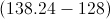and divide by the price before tax.  This gives 0.08 or 8%.

A certain state charges sales tax at a rate of 8.75% per dollar spent. John spends $127.50 total, including tax, on clothes. What was the total price of the clothing before tax was added? Possible Answers:Correct answer:Explanation: Since we know the total price with tax, we simply need to work backwards to remove the tax from the total price and find the price of the clothing itself. This can be done as follows: Clothing Price or C = Total price / 1 + tax rate C =$127.50/(1 + 0.0875) = $117.24 ### Example Question #1 : How To Find The Amount Of Sales Tax Jack is at the grocery store. He buys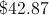in groceries, and groceries are not taxed in his state. He also buys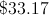in other items, which are taxed at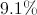(not yet applied). How much is his total? Possible Answers: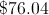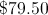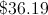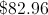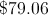Correct answer:Explanation: Jack's groceries are not taxed, so they cost, but his other items are taxed. We will calculate the price with the tax: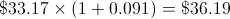We will add the two together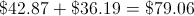### Example Question #1 : How To Find The Amount Of Sales Tax Harry, on his first lunch date with Sally, decides to put the entire bill on his credit card. If the bill came to$28 and Harry wants to leave an 18% tip, what is the total amount that he should pay?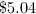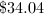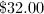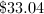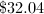Explanation:

To find an 18% increase, multiply by 1.18.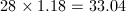### Example Question #1 : How To Find Simple Interest

Dan took out a $1200 loan at a rate of 3% simple interest a year. What is the amount of interest accrued for one month? Possible Answers:Correct answer:Explanation:$1200 is the amount that he took out.

3% annually would yield an interest amount of $36. Therefore, each month, he would be paying$3 a month.

### Example Question #1 : How To Find Simple Interest

Peter wanted to buy a car and borrowed $2,500 from the bank at 5% simple interest per year. He was able to pay back the loan in one lump sum at six months. What was the total amount he paid the bank? Possible Answers: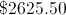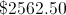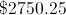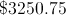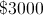Correct answer:Explanation: Simple interest is given by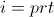where= interest,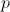= principal,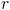= rate, and= time in years.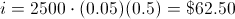Remember, six months is the same as half of a year. The total paid back to the bank is the principle plus the interest, or$2,562.50.

I have $100 in my bank. After one year, I have withdrawn a total of$20 and I still have $85 in the bank. What is the yearly interest rate of my bank account? Possible Answers:Correct answer:Explanation: Since I have withdrawn$20, that means I only have $80 in my account.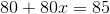imples that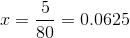which is 6.25%. ### Example Question #1 : Interest You really want to buy a car that costs$15,000 but you only have $5,000 saved. Rather than getting a bank loan, your parents offer to lend you the extra money but will require you to pay them back with 4.3% interest. How much will car cost in total after you have paid your parents back? Possible Answers: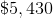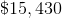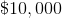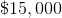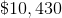Correct answer:Explanation: Amount borrowed =$15,000  $5,000 =$10,000

Interest of 4.3% = $10,000 * 0.043 =$430

Total cost of car = $5000 +$10000 + $430 =$15,430

### All PSAT Math Resources